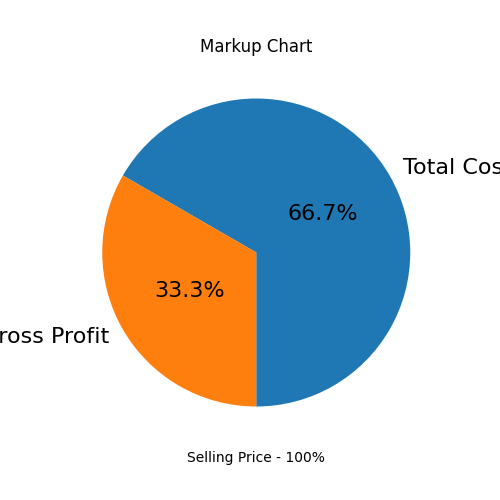×
EVEN THE mini TOOLS CAN EMPOWER PEOPLE TO DO GREAT THINGS.

# Markup Calculator

 Selling Price: Total Cost:
Markup Percentage = (Selling price - Total cost) / Total cost = Gross Profit / Total cost =
(150 - 100)/100 = 50/100 = 50%
Gross Profit = \$50If you have websites, you can embed this tool to increase your visitors' engagement. Or please share the result via:

The Markup Calculator is used to calculate the markup percent, which is the proportion of total cost represented by profit. The calculation is based on a product’s selling price and total cost.

## Formula

The markup percentage calculation formula is as follows:

Markup percentage = (Selling price - Total cost) / Total cost

### Frequently Used Miniwebtools:

•••••••••••••••••••×

### Do us a favor and answer 3 quick questions

Thank you for participating in our survey. Your input will help us to improve our services.

Where exactly did you first hear about us?

What is your favorite tool on our site?